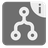Merge Intervals (iterator version)
Undefined
English

You are given a sequence of intervals, as iterator of the tuples of ints.
It is your task to minimize the number of intervals by merging those that intersect or by removing intervals fitting into another one. After that you have to create an iterator object which is linked to this list.

Since the range of values for the intervals is restricted to integers, you must also merge those intervals between which no value can be found.

An example:
Let's say you've got these 5 intervals: 1..6, 3..5, 7..10, 9..12 and 14..16.

1. 1..6 and 3..5
3..5 is a part of 1..6, so 3..5 must disappear.
2. 1..6 and 7..10
Even though the intervals don’t intersect, there isn’t an int type value between them, so they have to be merged with the new interval 1..10.
3. 1..10 and 9..12
These intervals do intersect, because 9 < 10, so they have to be merged with the new interval 1..12.
4. 1..12 and 14..16
These two intervals don’t intersect, so they mustn’t be merged.
So the intervals to be returned are:
1..12 and 14..16

Input: A sequence of intervals as an iterator of the tuples of 2 ints.

Output: The iterator object linked to the list of merged intervals.

Examples:

```list(merge_intervals(iter([(1, 4), (2, 6), (8, 10), (12, 19)]))) == [(1, 6), (8, 10), (12, 19)]
list(merge_intervals(iter([(1, 12), (2, 3), (4, 7)]))) == [(1, 12)]
list(merge_intervals(iter([(1, 5), (6, 10), (10, 15), (17, 20)]))) == [(1, 15), (17, 20)]```